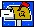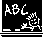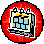CS 5314 Randomized Algorithms (Fall 2011)

General Information

 Lecturer:   Wing-Kai Hon (韓永楷)wkhon @ cs.nthu.edu.tw

 Tutor:   Wisely Ku   (古宗翰)       Bay-Yuan Hsu   (許倍源)thku @ cs.nthu.edu.tw bayyuan @ cs.nthu.edu.tw

 Meeting Times:Mon 1520—1710, Thu 1410—1500

 Announcement Recent Events Jan 05:   HW 5 Deadline + Solution  Jan 09:   Final Exam (Notes 21 to 23, HW 4)

 Dec 26: Lecture Notes 17, 18, and 19 posted Dec 29: Homework 5 (optional) releasedCourse Materials

 Lecture Topics Related Files 0 Overview [pdf] 1 Basics of Probability Theory [pdf] 2 Verifying Polynomial Identities [pdf] 3 Verifying Matrix Multiplication, Randomized Min-Cut [pdf] 4 Binomial Random Variables [pdf] 5 Geometric Random Variables, Conditional Expectation [pdf] 6 Coupon Collector Problem, Analysis of Quicksort [pdf] 7 Markov Inequality, Variance [pdf] 8 Common Variance, Chebyshev Inequality [pdf] 9 Randomized Median [pdf] 10 Chernoff Bounds: Introduction [pdf] 11 Chernoff Bounds: Application [pdf] 12 Chernoff Bounds: More Application [pdf]

 13 Balls, Bins, and Random Graphs (Balls-and-Bins Model) [pdf] 14 Balls, Bins, and Random Graphs (Poisson Approximation) [pdf] 15 Balls, Bins, and Random Graphs (Hashing) [pdf]

 17 Probabilistic Method (Introduction) [pdf] 18 Probabilistic Method (De-randomization, Sample and Modify) [pdf] 19 Probabilistic Method (2nd Moment, Conditional Expectation Inequality) [pdf]

 21 Markov Chains (Definition, Solving 2SAT) [pdf] 22 Markov Chains (Solving 3SAT) [pdf] 23 Markov Chains (Gambler’s Ruin, Random Walk) [pdf] 24 Markov Chains (Parrondo’s Paradox) [pdf]

 Tutorial Topics Related Files 1 Homework 1 Hints, Secretary Problem [pdf] 2 Homework 1 Solutions, Homework 2 Hints [ppt] 3 Homework 2 Solutions [ppt]

 Assignment Topics Related Files 1 Random Variables and Expectations [pdf] 2 Moments and Deviations [pdf] 3 Chernoff Bounds, Balls-and-Bins [pdf] 4 Markov Chains [pdf] 5 Probabilistic Methods [pdf]Teaching Plan

 1 Events and Probability Lecture 1 to Lecture 3 2 Discrete Random Variables and Expectation Lecture 4 to Lecture 6 3 Moments and Deviations Lecture 7 to Lecture 9 4 Chernoff Bounds Lecture 10 to Lecture 12 5 Balls, Bins, and Random Graphs Lecture 13 to Lecture 15 6 The Probabilistic Method Lecture 17 to Lecture 19 7 Markov Chain and Random Walk Lecture 21 to Lecture 24

Last updated:  December 29, 2011